CBSE Class 10 Sample Paper for 2023 Boards - Maths Standard

Class 10
Solutions of Sample Papers for Class 10 Boards

## (ii) volume of each new solid formed.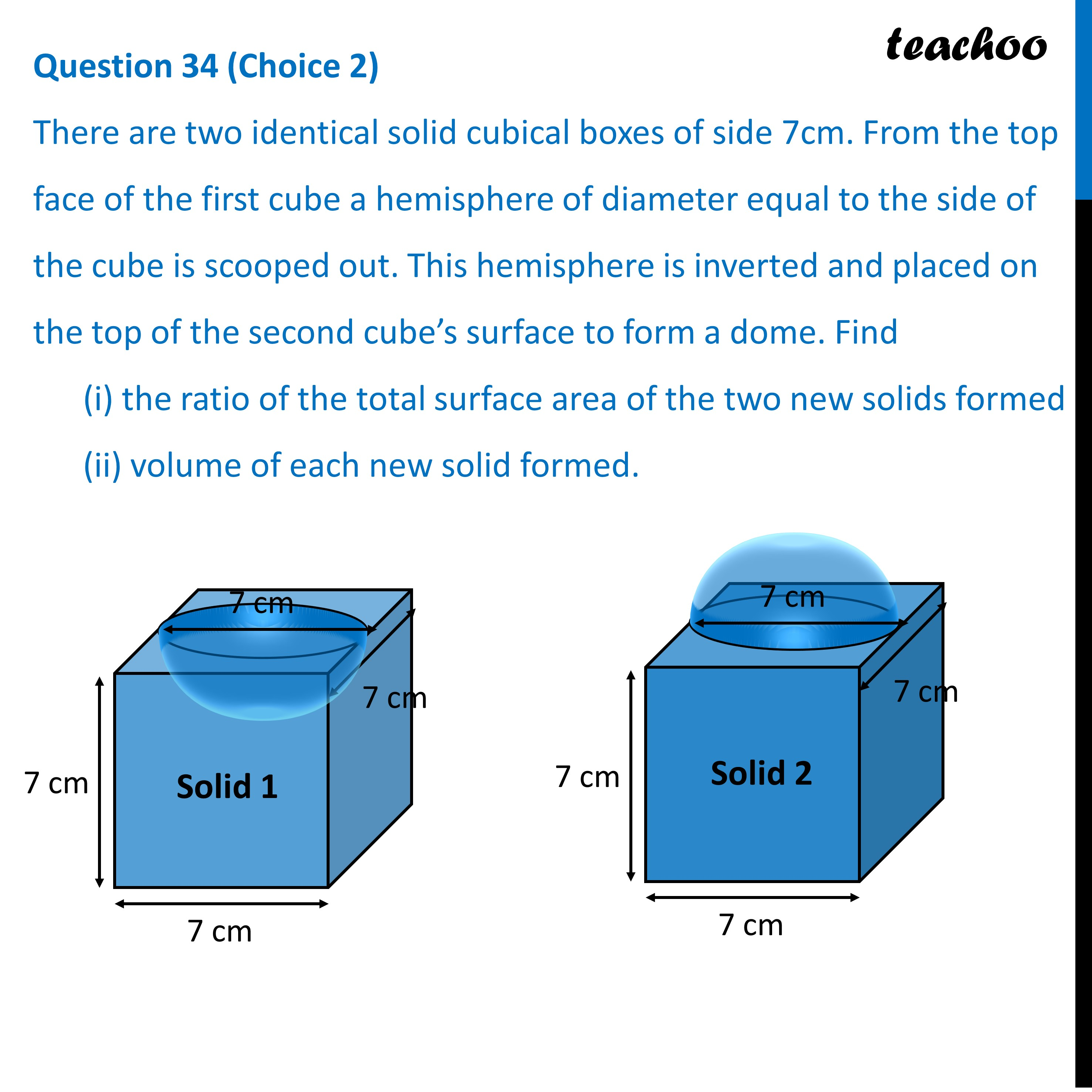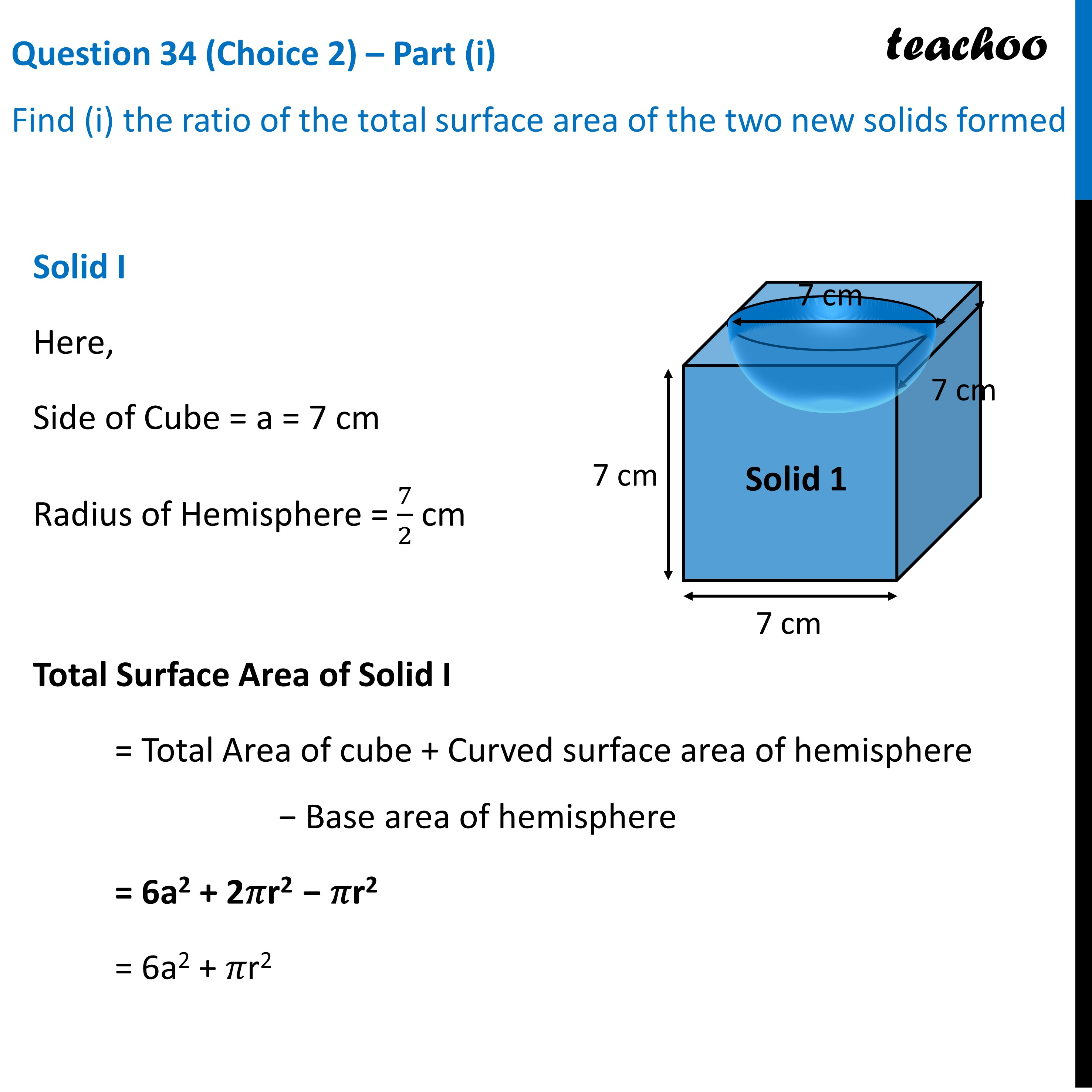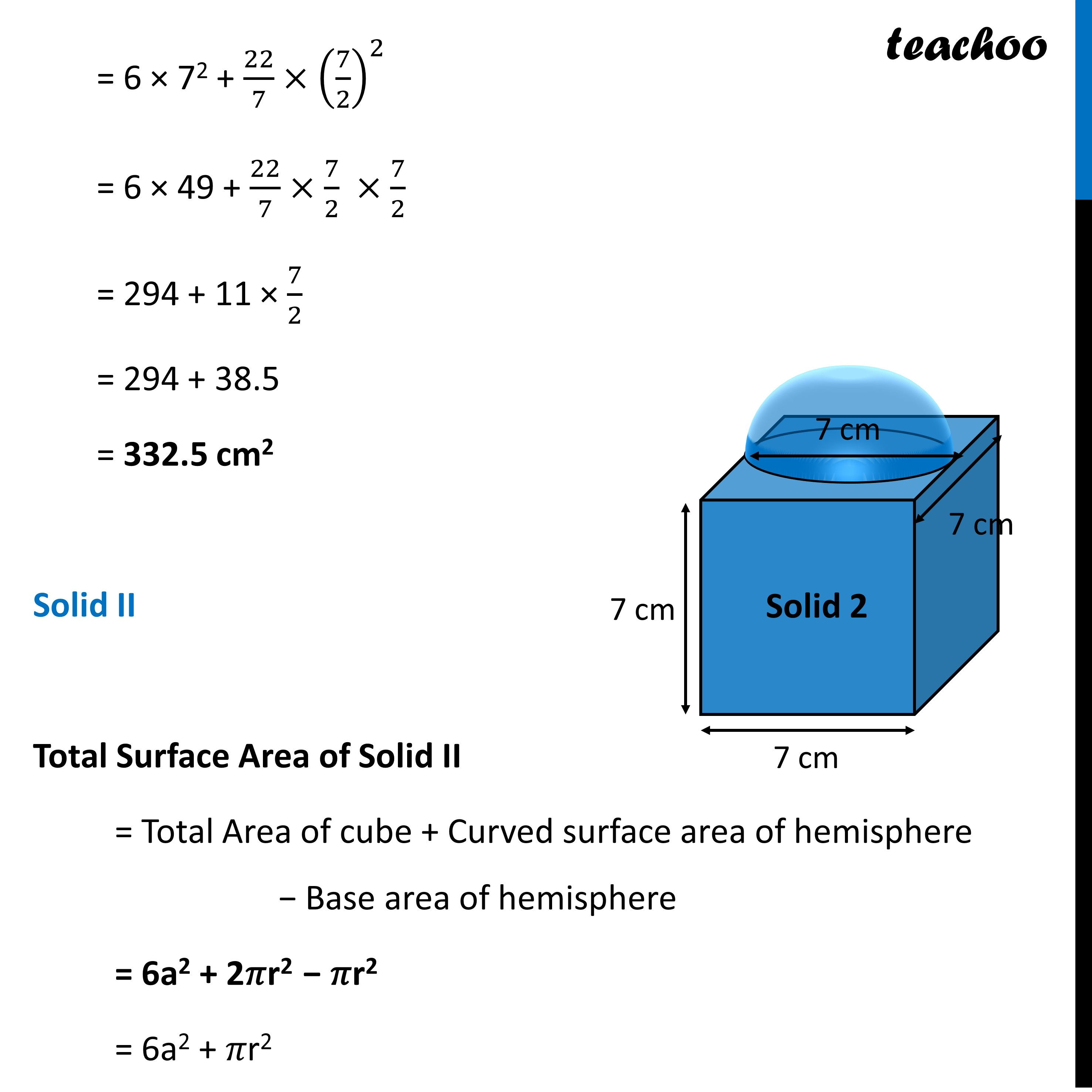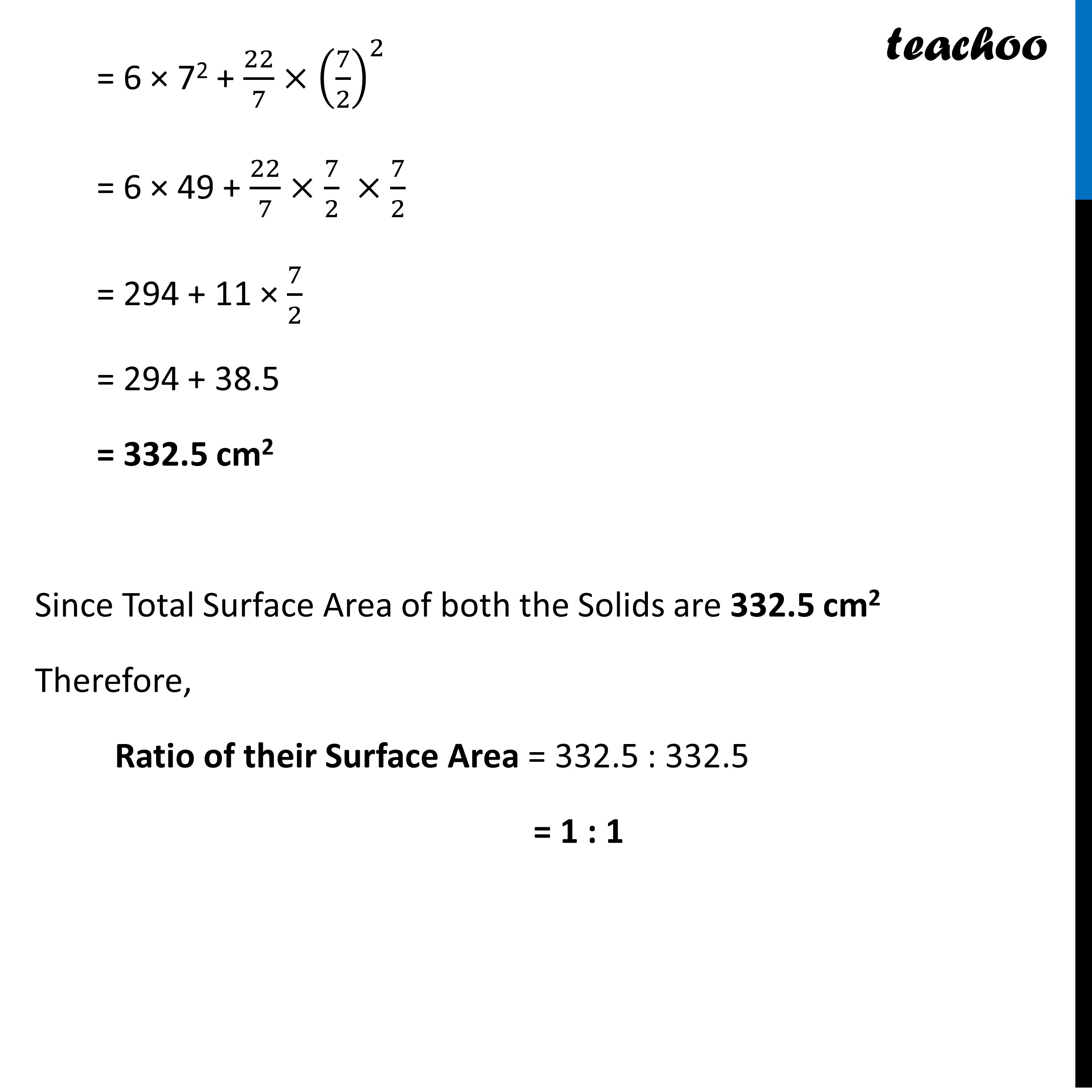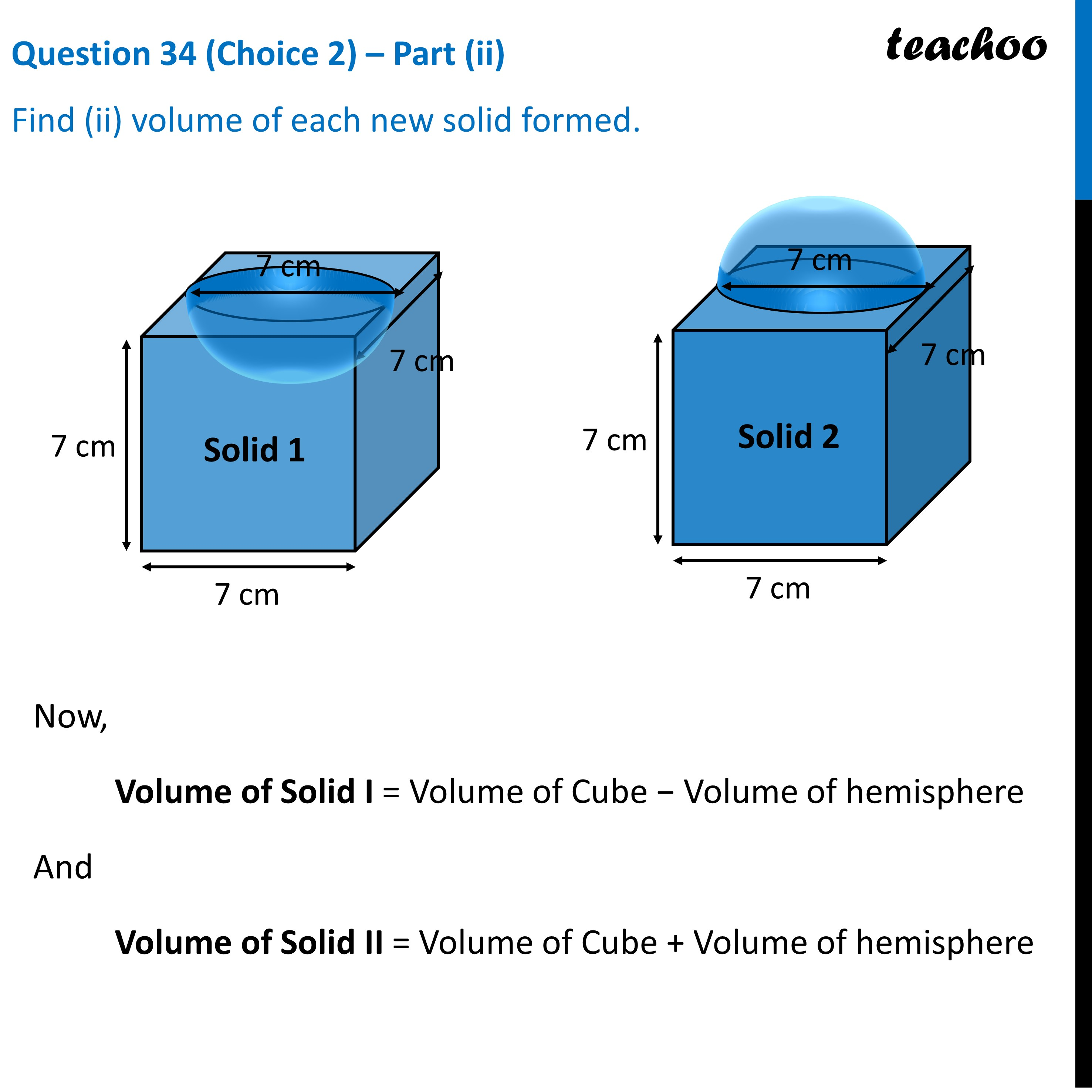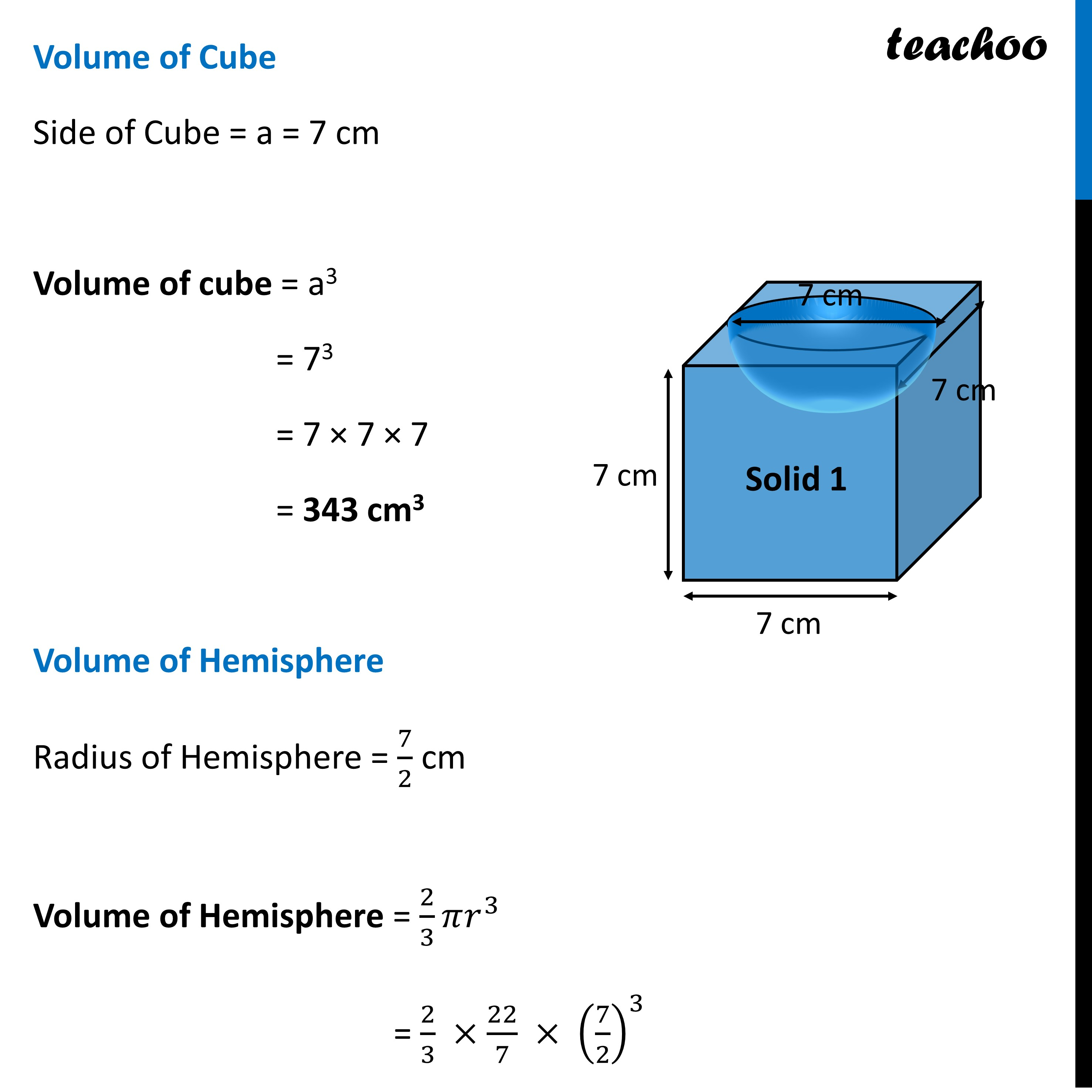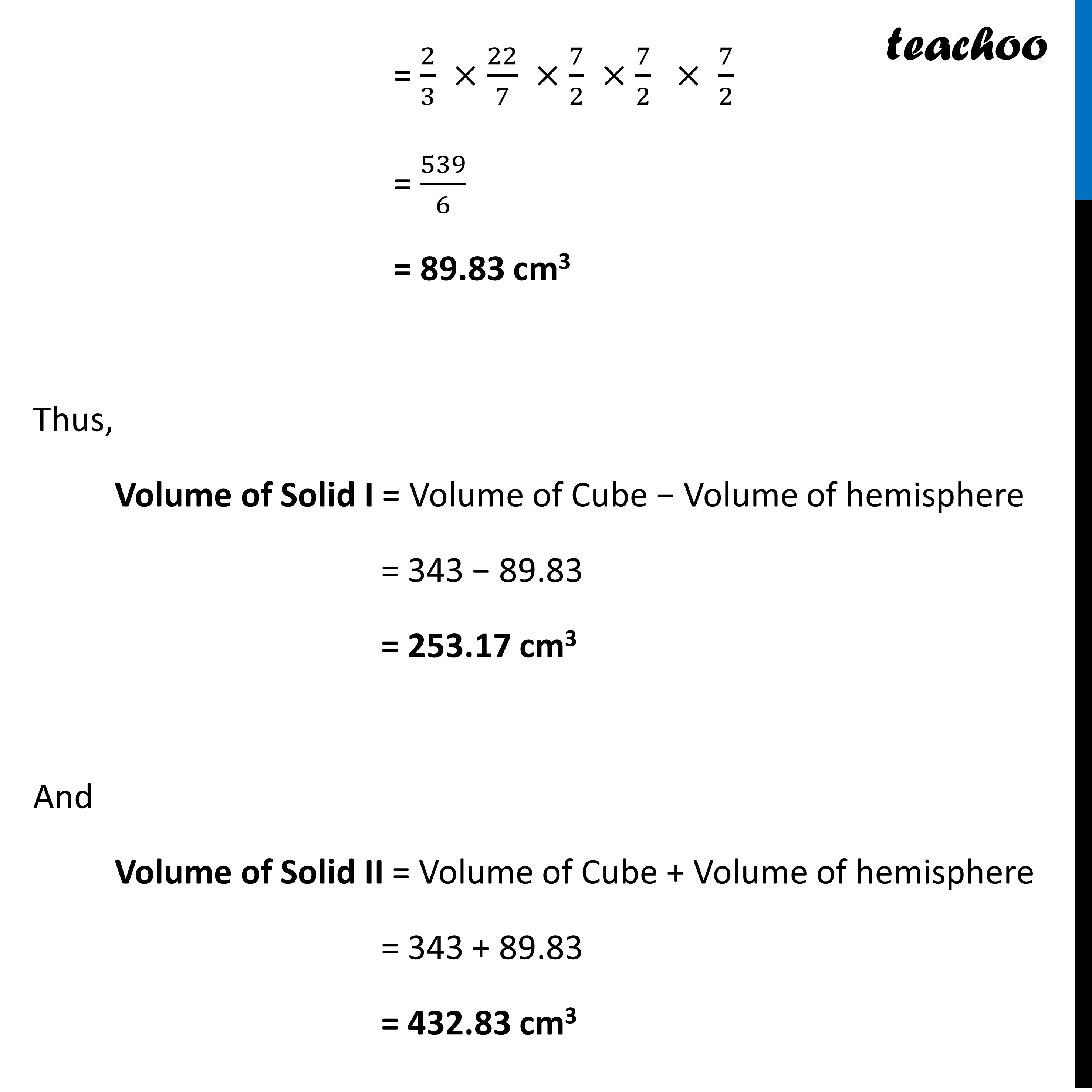Learn in your speed, with individual attention - Teachoo Maths 1-on-1 Class

### Transcript

Question 34 (Choice 2) There are two identical solid cubical boxes of side 7cm. From the top face of the first cube a hemisphere of diameter equal to the side of the cube is scooped out. This hemisphere is inverted and placed on the top of the second cube’s surface to form a dome. Find (i) the ratio of the total surface area of the two new solids formed (ii) volume of each new solid formed. Question 34 (Choice 2) – Part (i) Find (i) the ratio of the total surface area of the two new solids formed Solid I Here, Side of Cube = a = 7 cm Radius of Hemisphere = 7/2 cm Total Surface Area of Solid I = Total Area of cube + Curved surface area of hemisphere − Base area of hemisphere = 6a2 + 2𝜋r2 − 𝜋r2 = 6a2 + 𝜋r2 = 6 × 72 + 22/7×(7/2)^2 = 6 × 49 + 22/7×7/2 ×7/2 = 294 + 11 × 7/2 = 294 + 38.5 = 332.5 cm2 Solid II Total Surface Area of Solid II = Total Area of cube + Curved surface area of hemisphere − Base area of hemisphere = 6a2 + 2𝜋r2 − 𝜋r2 = 6a2 + 𝜋r2 = 6 × 72 + 22/7×(7/2)^2 = 6 × 49 + 22/7×7/2 ×7/2 = 294 + 11 × 7/2 = 294 + 38.5 = 332.5 cm2 Since Total Surface Area of both the Solids are 332.5 cm2 Therefore, Ratio of their Surface Area = 332.5 : 332.5 = 1 : 1Question 34 (Choice 2) – Part (ii) Find (ii) volume of each new solid formed.Now, Volume of Solid I = Volume of Cube − Volume of hemisphere And Volume of Solid II = Volume of Cube + Volume of hemisphere Volume of Cube Side of Cube = a = 7 cm Volume of cube = a3 = 73 = 7 × 7 × 7 = 343 cm3 Volume of Hemisphere Radius of Hemisphere = 7/2 cm Volume of Hemisphere = 2/3 𝜋𝑟^3 = 2/3 ×22/7 × (7/2)^3 = 2/3 ×22/7 ×7/2 ×7/2 × 7/2 7/2= 2/3 ×22/7 ×7/2 ×7/2 × 7/2 = 539/6 = 89.83 cm3 Thus, Volume of Solid I = Volume of Cube − Volume of hemisphere = 343 − 89.83 = 253.17 cm3 And Volume of Solid II = Volume of Cube + Volume of hemisphere = 343 + 89.83 = 432.83 cm3